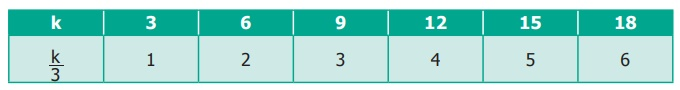Home | | Maths 6th Std | Exercise 2.2

# Exercise 2.2

6th Maths : Term 1 Unit 2 : Introduction To Algebra : Text Book Back Exercises Questions with Answers, Solution

Exercise 2.2

1. Fill in the blanks.

(i) The algebraic statement of ‘f ’ decreased by 5 is f − 5.

(ii) The algebraic statement of ‘s’ divided by 5 is s / 5.

(iii) The verbal statement of '2m − 10' is 10 less to 2 times ‘m’.

(iv) If A’s age is ‘n’ years now, 7 years ago A’s age was n − 7.

(v) If ‘p − 5’ gives 12 then ‘p’ is 17.

Soution: P − 5 = 12, P = 12 + 5, P = 17

2. Say True or False.

(i) 10 more to three times 'c' is '3c + 13' [False]

(ii) If the cost of 10 rice bags is  ‘t’, then the cost of 1 rice bag is  ‘t’/10 . [True]

(iii) The statements ‘x’ divided by 3 and 3 divided by‘x’ are the same. [False]

(iv) The product of ‘q’ and 20 is '20q'. [True]

(v) 7 less to 7 times ‘y’ is '7 − 7y'. [False]

3. Express the following verbal statement to algebraic statement.

(iii) 8 reduced by ‘y’. Answer: 8 − y

(v) 4 less to 9 times of ‘y’. Answer: 9y − 4

4. Express the following algebraic statement to verbal statement.

(i) x ÷ 3

(ii) 5n − 12

Answer: 12 less to 5 times n

(iii) 11 + 10x

(iv) 70s

Answer: product of 70 and s

5. The teacher asked two students to write the algebraic statement for the verbal statement “8 more than a number”. Vetri wrote ‘8 + x’ but Maran wrote ‘8x’. Who gave the correct answer?

(i) If ‘n’ takes the value 3 then find the value of ‘n + 10’ ?

Answer: n = 3; n + 10 = 3 + 10

= 13

(ii) If ‘g’ is equal to 300 what is the value of ‘g − 1’ and ‘g + 1’ ?

g = 300

g − 1 = 300 − 1 = 299

g + 1 = 300+ 1 = 301

(iii) What is the value of ‘s’, if '2s − 6' gives 30?

2s − 6 = 30

2s = 30 + 6

2s = 36

s = 36/2 =  18

s = 18

7. Complete the table and find the value of ‘k’ for which ‘k’/3 gives 5.Objective Type Questions

8. The value of ‘y’ in y + 7 = 13 is

(a) y = 5

(b) y = 6

(c) y = 7

(d) y = 8

Soution: y = 13 − 7 = 6

9. 6 less to ‘n’ gives 8 is represented as

(a) n − 6 = 8

(b) 6 − n = 8

(c) 8 − n = 6

(d) n − 8 = 6

Answer: (a) n − 6 = 8

10. The value of ‘c’ for which 3c/4  gives 18 is

(a) c = 15

(b) c = 21

(c) c = 24

(d) c = 27

Soution: 3c / 4 = 18

3C = 4 × 18 = 4×18 / 3

C = 4 × 6

C = 24

Exercise 2.2

1) (i) f − 5 (ii) s/5 (iii) 10 less to 2 times m  (iv) n − 7 (v) 17

2) (i) False (ii) True (iii) False (iv) True (v) False

3) (i) t + 100 (ii) 4q (iii) 8 − y (iv) 56 + 2x (v) 9y − 4

4) (i) x divided by 3 (ii) 12 less to 5 times n (iii) 11 added to 10 times x  (iv) product of 70 and s

6) (i) 13 (ii) 299; 301 (iii)18

7)The value of ‘k’ is 15.

8) b) y = 6

9) a) n − 6 = 8

10) c) c = 24

Tags : Questions with Answers, Solution | Introduction to Algebra | Term 1 Chapter 2 | 6th Maths , 6th Maths : Term 1 Unit 2 : Introduction To Algebra
Study Material, Lecturing Notes, Assignment, Reference, Wiki description explanation, brief detail
6th Maths : Term 1 Unit 2 : Introduction To Algebra : Exercise 2.2 | Questions with Answers, Solution | Introduction to Algebra | Term 1 Chapter 2 | 6th Maths